首页  >  原创  >  正文本周监管警告更新，以下平台一定注意远离！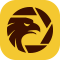外汇天眼   |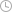2022-01-02 13:26:28

分享到：

摘要：监管信息早知道！外汇天眼 将每周定期公布监管牌照状态发生变化的交易商，以供投资者参考，规避投资风险。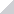监管信息早知道！外汇天眼 将每周定期公布监管牌照状态发生变化的交易商，以供投资者参考，规避投资风险。以下是监管牌照发生变动的交易商平台，注意警惕！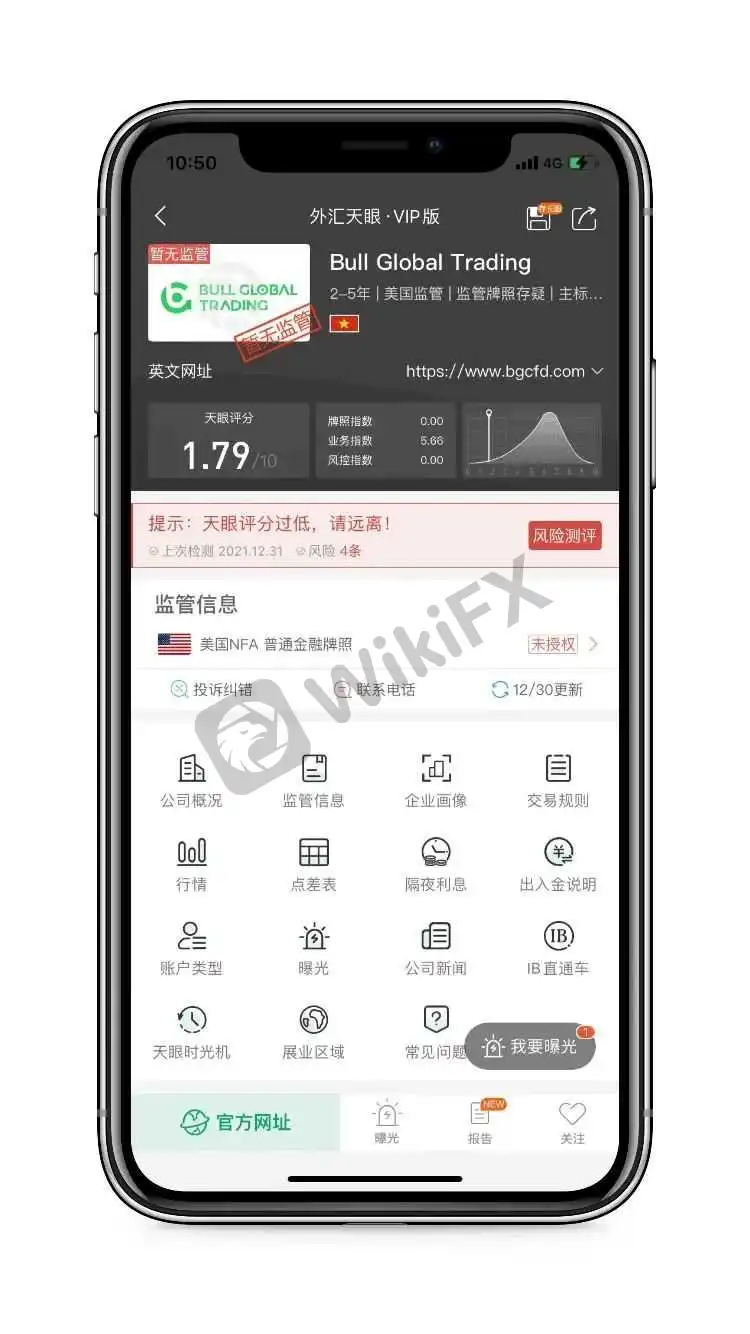Moore CAPITAL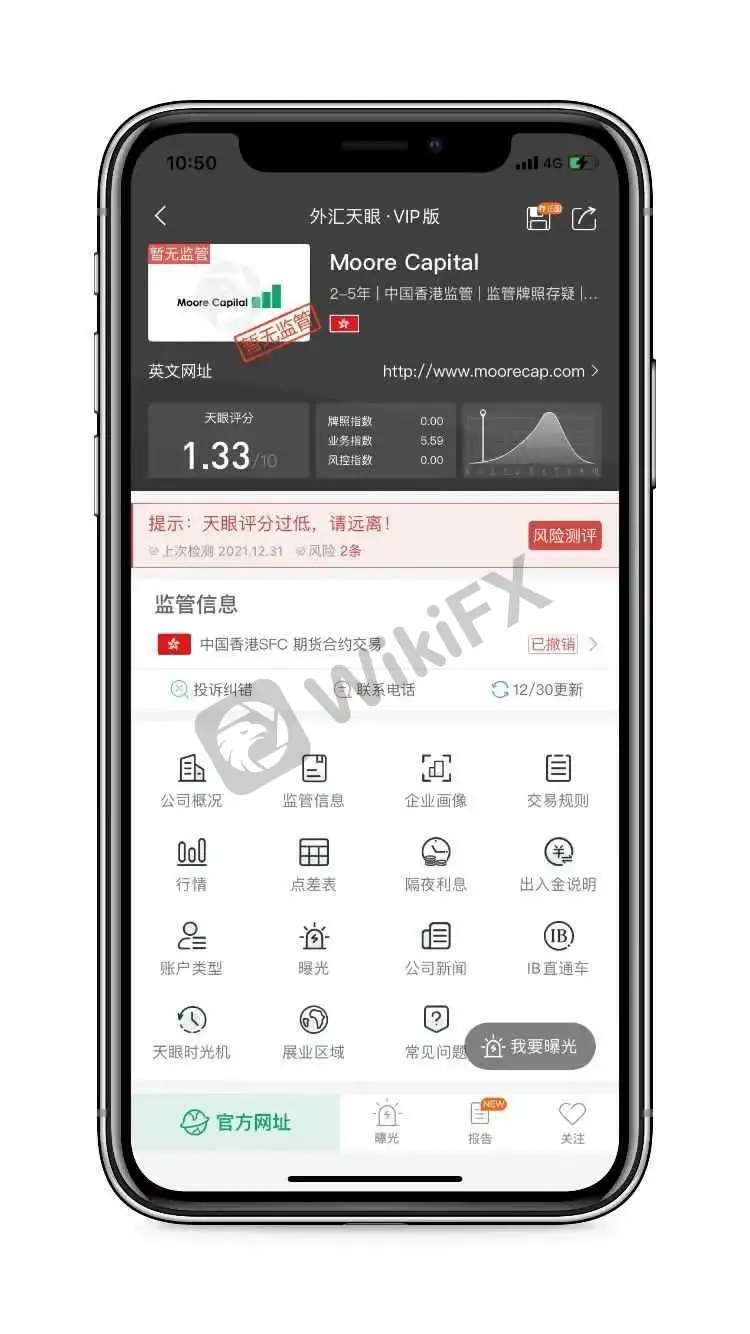Moore Capital，其宣称持有的中国香港SFC授权的期货合约交易牌照，从“监管中”变为“已撤销”。

Moore Capital，天眼评分1.33分，其宣称持有的牌照被撤销后，当前暂无任何实际有效的监管牌照，存在巨大风险，建议投资者们远离。人民币

• 美元
• 澳大利亚元
• 巴西真正
• 加拿大元
• 瑞士法郎
• 智利比索
• 人民币
• 捷克克朗
• 丹麦克罗钠
• 欧元
• 英镑
• 港币
• 匈牙利福林
• 印度尼西亚卢比
• 以色列新锡克尔
• 印度卢比
• 日元
• 韩元
• 墨西哥比索
• 马来西亚林吉特
• 挪威克朗
• 新西兰元
• 菲律宾比索
• 巴基斯坦卢比
• 波兰兹罗提
• 俄罗斯卢布
• 瑞典克朗
• 新加坡元
• 泰铢
• 土耳其里拉
• 新台币
• 南非兰特
• 阿联酋迪拉姆
• 保加利亚列弗
• 克罗地亚库纳
• 毛里求斯卢比
• 罗马尼亚列伊
• 冰岛克朗
• 尼日利亚奈拉
• 哥伦比亚比索
• 阿根廷比索
• 秘鲁索尔
• 越南盾
• 乌克兰格里夫纳
• 阿尔巴尼亚列克
• 亚美尼亚特拉姆
• 阿塞拜疆马纳特
• 波黑元
• 孟加拉塔卡
• 巴林丁那
• 百慕大美元
• 白俄罗斯卢布
• 哥斯大黎加科郎
• 古巴比索
• 阿尔及利亚丁那
• 埃及磅
• 格鲁吉亚拉里
• 加纳塞地
• 危地马拉格查尔
• 洪都拉斯伦皮拉
• 伊拉克丁那
• 伊朗里亚尔
• 牙买加元
• 约旦丁那
• 肯尼亚先令
• 吉尔吉斯斯坦索姆
• 柬埔寨瑞尔
• 科威特丁那
• 哈萨克斯坦坚戈
• 黎巴嫩镑
• 斯里兰卡卢比
• 摩洛哥 迪拉姆
• 摩尔多瓦列伊
• 马其顿第纳尔
• 缅甸元
• 蒙古图格里克
• 纳米比亚元
• 尼加拉瓜科多巴
• 尼泊尔卢比
• 阿曼里亚尔
• 巴拿马巴波亚
• 卡塔尔里亚尔
• 塞尔维亚第纳尔
• 沙特里亚尔
• 南苏丹镑
• 突尼斯第纳尔
• 乌干达先令
• 乌拉圭新比索
• 乌兹别克斯坦苏姆
• 委内瑞拉玻利瓦尔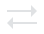• 美元
• 澳大利亚元
• 巴西真正
• 加拿大元
• 瑞士法郎
• 智利比索
• 人民币
• 捷克克朗
• 丹麦克罗钠
• 欧元
• 英镑
• 港币
• 匈牙利福林
• 印度尼西亚卢比
• 以色列新锡克尔
• 印度卢比
• 日元
• 韩元
• 墨西哥比索
• 马来西亚林吉特
• 挪威克朗
• 新西兰元
• 菲律宾比索
• 巴基斯坦卢比
• 波兰兹罗提
• 俄罗斯卢布
• 瑞典克朗
• 新加坡元
• 泰铢
• 土耳其里拉
• 新台币
• 南非兰特
• 阿联酋迪拉姆
• 保加利亚列弗
• 克罗地亚库纳
• 毛里求斯卢比
• 罗马尼亚列伊
• 冰岛克朗
• 尼日利亚奈拉
• 哥伦比亚比索
• 阿根廷比索
• 秘鲁索尔
• 越南盾
• 乌克兰格里夫纳
• 阿尔巴尼亚列克
• 亚美尼亚特拉姆
• 阿塞拜疆马纳特
• 波黑元
• 孟加拉塔卡
• 巴林丁那
• 百慕大美元
• 白俄罗斯卢布
• 哥斯大黎加科郎
• 古巴比索
• 阿尔及利亚丁那
• 埃及磅
• 格鲁吉亚拉里
• 加纳塞地
• 危地马拉格查尔
• 洪都拉斯伦皮拉
• 伊拉克丁那
• 伊朗里亚尔
• 牙买加元
• 约旦丁那
• 肯尼亚先令
• 吉尔吉斯斯坦索姆
• 柬埔寨瑞尔
• 科威特丁那
• 哈萨克斯坦坚戈
• 黎巴嫩镑
• 斯里兰卡卢比
• 摩洛哥 迪拉姆
• 摩尔多瓦列伊
• 马其顿第纳尔
• 缅甸元
• 蒙古图格里克
• 纳米比亚元
• 尼加拉瓜科多巴
• 尼泊尔卢比
• 阿曼里亚尔
• 巴拿马巴波亚
• 卡塔尔里亚尔
• 塞尔维亚第纳尔
• 沙特里亚尔
• 南苏丹镑
• 突尼斯第纳尔
• 乌干达先令
• 乌拉圭新比索
• 乌兹别克斯坦苏姆
• 委内瑞拉玻利瓦尔
当前汇率  ：
--
请输入金额
人民币
可兑换金额
-- 美元

猜你喜欢

分享到微信朋友圈 ×
打开微信，点击底部的“发现”，
使用“扫一扫”即可将网页分享至朋友圈
• 微信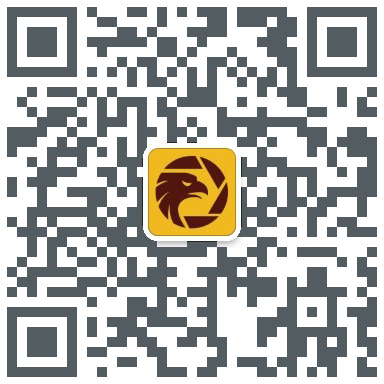• QQ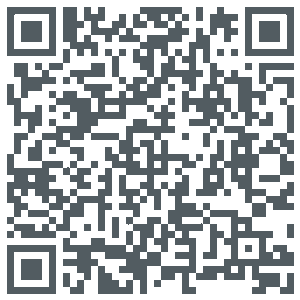• APP• 置顶